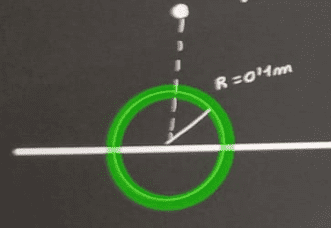# Surface charge density of a plane

Guillem_dlc
Homework Statement:
A positive point charge of value ##q=+2\, \mu \textrm{C}## is located at coordinate point ##(0,0,5)\, \textrm{cm}##, above an infinite homogeneously charged plane located at ##z=0##. If the flux through a sphere of radius ##R=10\, \textrm{cm}## centered at the origin of coordinates is ##1,08\cdot 10^5\, \textrm{Nm}^2/\textrm{C}##, calculate the value of the surface charge density of the plane.
Relevant Equations:
Gauss's Law
$$\phi_E=\dfrac{Q_{\textrm{enclosed}}}{\varepsilon_0}\Rightarrow Q_{\textrm{enclosed}}=9,6\cdot 10^{-7}\, \textrm{C}$$
$$Q_{\textrm{enclosed}}=\sigma S=\sigma \pi R^2\Rightarrow \sigma =\dfrac{Q_{\textrm{enclosed}}}{\pi (0,1^2)}=3,04\cdot 10^{-5}\, \textrm{C}/\textrm{m}^2$$

I have a lot of problems with the flux exercises. I have a hard time seeing how they act when I have more than one element in the system as in this case.

Last edited:

Gordianus
Can you draw the point charge, the plane and the sphere?

Guillem_dlc
Can you draw the point charge, the plane and the sphere?Staff Emeritus
Homework Helper
Gold Member
You have drawn the point charge outside of the sphere. Is this accurate according to the problem formulation?

Guillem_dlc
You have drawn the point charge outside of the sphere. Is this accurate according to the problem formulation?
No, I see now. I think that I have the solution:

$$\phi_E = \dfrac{Q_{\textrm{enclosed}}}{\varepsilon_0} \Rightarrow Q_{\textrm{enclosed}}=9,6\cdot 10^{-7}\, \textrm{C}$$
$$\phi_{\textrm{enclosed}}=\sigma S=\sigma \pi R^2+q\Rightarrow \sigma=\dfrac{Q_{\textrm{enclosed}}-q}{S}=-33\, \mu \textrm{C}/\textrm{m}^2$$

Gordianus
A good drawing fixes many problems.